Home | | Maths 12th Std | Choose the correct Answers

## Chapter: 12th Mathematics : UNIT 2 : Complex Numbers

Choose the correct or the most suitable answer from the given four alternatives, Multiple choice questions with Answers, Solution - Maths Book back 1 mark questions and answers with solution for Exercise Problems

Choose the correct or the most suitable answer from the given four alternatives :

1. i+ in++ in++ in+is

(1) 0

(2) 1

(3) ã1

(4) i2. The value ofis

(1) 1+ i

(2) i

(3) 1

(4) 03 .The area of the triangle formed by the complex numbers ziz, and + iz in the Argandãs diagram is

(1) 1/2 | z |2

(2) | z |2

(3) 3/2 | z |2

(4) 2 | z |2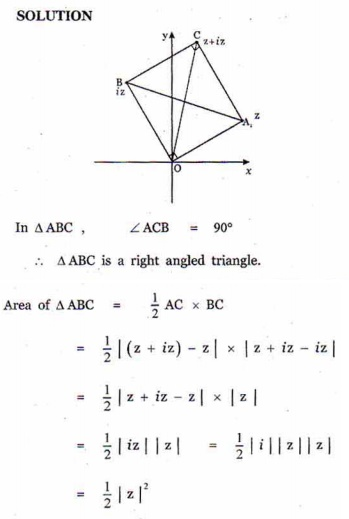4. The conjugate of a complex number is 1/i-2 Then, the complex number is

(1) 1/[i+2]

(2) -1/[i+2]

(3) -1/[i-2]

(4) 1/[i-2]5. If z=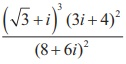then | | is equal to

(1) 0

(2) 1

(3) 2

(4) 36. If is a non zero complex number, such that 2iz=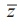then | | is

(1) 1/2

(2) 1

(3) 2

(4) 37. If | ã 2 + ãÊ 2 , then the greatest value of | | is

(1) ã3 ã 2

(2) ã3 + 2

(3) ã5 ã 2

(4) ã5 + 28. If, then the least value of | | is

(1) 1

(2) 2

(3) 3

(4) 5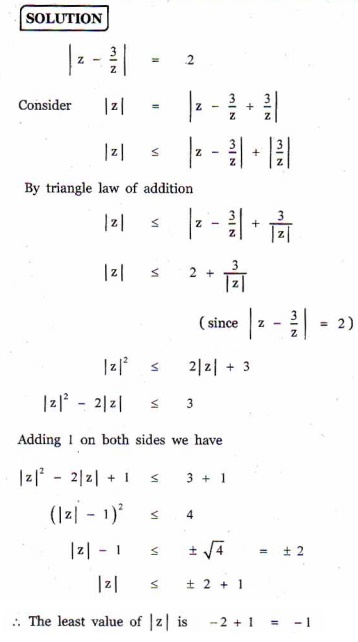9. If | = 1, then the value ofis

(1) z

(2)(3) 1/z

(4) 110. The solution of the equation | ã = 1+ 2is

(1) (3/2) ã 2i

(2) ã  (3/2) + 2i

(3) 2 ã (3/2)i

(4) 2 + (3/2) i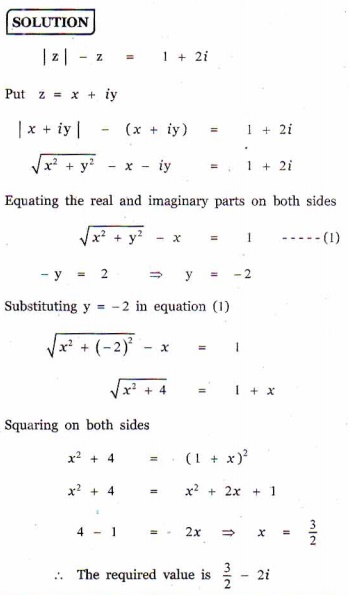11. If | z= 1, | z= 2, | z= 3 and | 9zz+ 4zz+ zz= 12 , then the value of | z+ z+ z| is

(1) 1

(2) 2

(3) 3

(4) 412. If is a complex number such that ã R and + 1/z ã R , then | | is

(1) 0

(2) 1

(3) 2

(4) 313. zz, and zare complex numbers such that z+ z+ z= 0 and | z=z=z= 1 then + + is

(1) 3

(2) 2

(3) 1

(4) 014. If (z-1) / (z+1), is purely imaginary, then | | is

(1) 1/2

(2) 1

(3) 2

(4) 315. If = + iy is a complex number such that | + 2 | = | ã 2 | , then the locus of is

(1) real axis

(2) imaginary axis

(3) ellipse

(4) circle16. The principal argument of 3/(-1+i ) is

(1) -5ü/6

(2) -2ü/3

(3) -3ü/4

(4) -ü/217. The principal argument of (sin 40ô¯+ cos 40ô¯)5  is

(1) ã110ô¯

(2) ã70ô¯

(3) 70ô¯

(4) 110ô¯18.If (1+ i) (1+ 2i) (1+ 3i)L(1+ ni= + iy , then 2 ã 5 ã10L(1+ n) is

(1) 1

(2) i

(3) x2 + y2

(4) 1 + n219. If ü ã  1 is a cubic root of unity and (1+ ü)= + Bü , then ( AB) equals

(1) (1, 0)

(2) ( ã1, 1)

(3) ( 0, 1)

(4) (1, 1)20. The principal argument of the complex number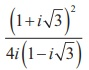is

(1) 2ü/3

(2) ü/6

(3) 5ü/6

(4) ü/2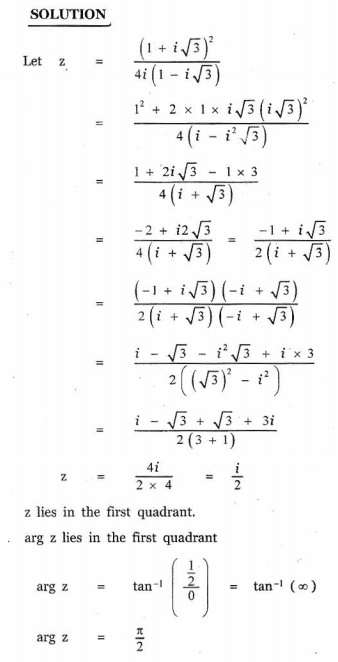21. If öÝ and öý are the roots of x+ += 0 , then öÝ 2020 + öý 2020 is

(1) ã2

(2) ã1

(3) 1

(4) 222. The product of all four values ofis

(1) ã2

(2) ã1

(3) 1

(4) 2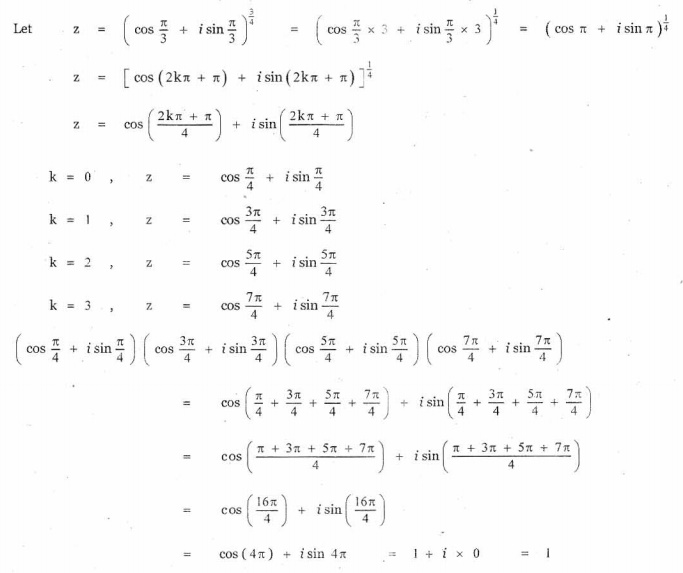23. If ü ã  1 is a cubic root of unity and= 3k then is equal to

(1) 1

(2) ã1

(3) ã3i

(4) ã ã3i24. The value of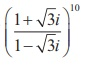isAns: (1)25.If ü = cis (2ü/3),  then the number of distinct  roots of(1) 1

(2) 2

(3) 3

(4) 4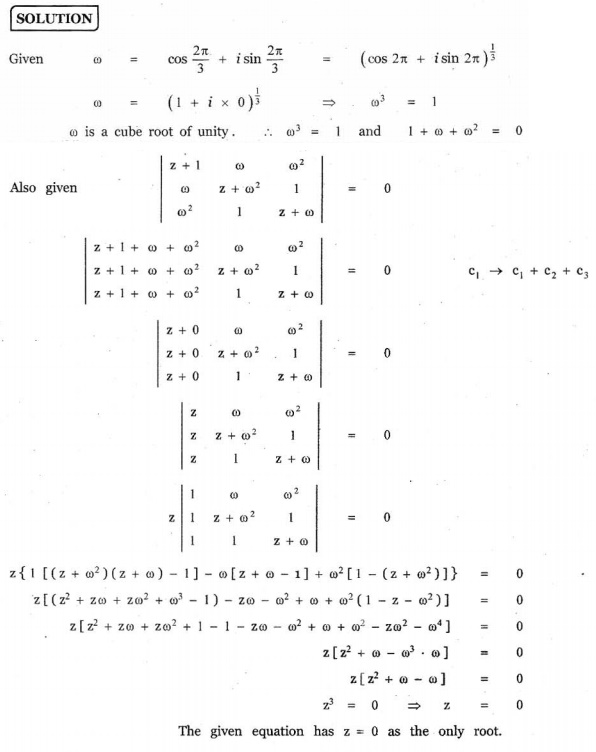Choose the correct or the most suitable answer from the given four alternatives :

1. i+ in++ in++ in+is

(1) 0

(2) 1

(3) ã1

(4) i

2. The value ofis

(1) 1+ i

(2) i

(3) 1

(4) 0

3 .The area of the triangle formed by the complex numbers ziz, and + iz in the Argandãs diagram is

(1) 1/2 | z |2

(2) | z |2

(3) 3/2 | z |2

(4) 2 | z |2

4. The conjugate of a complex number is 1/i-2 Then, the complex number is

(1) 1/[i+2]

(2) -1/[i+2]

(3) -1/[i-2]

(4) 1/[i-2]

5. If z=then | | is equal to

(1) 0

(2) 1

(3) 2

(4) 3

6. If is a non zero complex number, such that 2iz=then | | is

(1) 1/2

(2) 1

(3) 2

(4) 3

7. If | ã 2 + ãÊ 2 , then the greatest value of | | is

(1) ã3 ã 2

(2) ã3 + 2

(3) ã5 ã 2

(4) ã5 + 2

8. If, then the least value of | | is

(1) 1

(2) 2

(3) 3

(4) 5

9. If | = 1, then the value ofis

(1) z

(2)(3) 1/z

(4) 1

10. The solution of the equation | ã = 1+ 2is

(1) (3/2) ã 2i

(2) ã  (3/2) + 2i

(3) 2 ã (3/2)i

(4) 2 + (3/2) i

11. If | z= 1, | z= 2, | z= 3 and | 9zz+ 4zz+ zz= 12 , then the value of | z+ z+ z| is

(1) 1

(2) 2

(3) 3

(4) 4

12. If is a complex number such that ã R and + 1/z ã R , then | | is

(1) 0

(2) 1

(3) 2

(4) 3

13. zz, and zare complex numbers such that z+ z+ z= 0 and | z=z=z= 1 then + + is

(1) 3

(2) 2

(3) 1

(4) 0

14. If (z-1) / (z+1), is purely imaginary, then | | is

(1) 1/2

(2) 1

(3) 2

(4) 3

15. If = + iy is a complex number such that | + 2 | = | ã 2 | , then the locus of is

(1) real axis

(2) imaginary axis

(3) ellipse

(4) circle

16. The principal argument of 3/(-1+i ) is

(1) -5ü/6

(2) -2ü/3

(3) -3ü/4

(4) -ü/2

17. The principal argument of (sin 40ô¯+ cos 40ô¯)5  is

(1) ã110ô¯

(2) ã70ô¯

(3) 70ô¯

(4) 110ô¯

18.If (1+ i) (1+ 2i) (1+ 3i)L(1+ ni= + iy , then 2 ã 5 ã10L(1+ n) is

(1) 1

(2) i

(3) x2 + y2

(4) 1 + n2

19. If ü ã  1 is a cubic root of unity and (1+ ü)= + Bü , then ( AB) equals

(1) (1, 0)

(2) ( ã1, 1)

(3) ( 0, 1)

(4) (1, 1)

20. The principal argument of the complex numberis

(1) 2ü/3

(2) ü/6

(3) 5ü/6

(4) ü/2

21. If öÝ and öý are the roots of x+ += 0 , then öÝ 2020 + öý 2020 is

(1) ã2

(2) ã1

(3) 1

(4) 2

22. The product of all four values ofis

(1) ã2

(2) ã1

(3) 1

(4) 2

23. If ü ã  1 is a cubic root of unity and= 3k then is equal to

(1) 1

(2) ã1

(3) ã3i

(4) ã ã3i

24. The value ofisAns: (1)

25.If ü = cis (2ü/3),  then the number of distinct  roots of(1) 1

(2) 2

(3) 3

(4) 4Tags : Complex Numbers , 12th Mathematics : UNIT 2 : Complex Numbers
Study Material, Lecturing Notes, Assignment, Reference, Wiki description explanation, brief detail
12th Mathematics : UNIT 2 : Complex Numbers : Choose the correct Answers | Complex Numbers